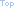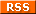# Dr. Brian Robert Callahan

academic, developer, with an eye towards a brighter techno-social life

## 2020-11-18Comparing a new language for tiny machines

You can see all the code (and more) for this blog post here.

I've always been interested in compilers and languages that are entirely developed by a single person or a small team. Obviously, I wouldn't want to give up our LLVMs and our GCCs. But I also think there's something nice about a compiler or a language developed by the smallest of teams. Astute readers might remember an earlier blog post in which I compared binary sizes for our SnakeQR game with a variety of different C compilers. One of those compilers was lacc, a C compiler developed entirely by a single person.

Recently, I became aware of a Cowgol, a programming language designed for 8-bit CPUs. The idea here is to develop a language that can have its toolchain be self-hosting on those 8-bit CPUs. This obviously means many small independent passes, so that each pass can be implemented as a stand-alone binary and those binaries will stay small enough to fit on those 8-bit systems and compile meaningful code on those systems. But Cowgol also has the benefit of having a generic C backend, allowing for easy bootstrapping, with the added benefit of Cowgol being a viable language for any machine that has a native C compiler (of course, Cowgol is far from the first language to transpile to C). It also means that Cowgol is a language that ideally is a write once, compile for systems as varied as CP/M on an Intel 8080 (or Zilog Z80) up to and including our modern Unix systems like OpenBSD all using a single, very quick to compile, toolchain. Neat.

Upstream seemed surprised that I was writing real programs in Cowgol, so I'm led to believe I might be the only one using the language. But that's fine. Let's write a simple program in C and in Cowgol so that we can compare them. Even if you're not going to use Cowgol, comparing programming languages I find to be a fun exercise and it might help you think about your own language choices, no matter what languages you choose to use.

#### A simple program: a Reverse Polish notation calculator

Let's write a quick RPN calculator. It can be done relatively quickly and in about 100 lines of code. This may not be the best example of an RPN calculator ever: decisions were made so that the C code and the Cowgol code were as identical as possible and as a result follow the same general design choices, even if those aren't the best design choices. Let's chalk it up to the fact that Cowgol is a new language and may not have the same facilities as something like C. I also simply chose to cut corners like not being able to input negative numbers directly with a unary - so if you want for example a -3 you would have to input 0 3 - for that. Like I said, this blog post isn't about writing an amazing RPN calculator, it's about comparing Cowgol with C.

#### RPN in C

First, let's look at the C code for our RPN calculator. We'll look at C first since that's not the new language.

Nothing special here. You write your expression out on the command line as arguments to the RPN calculator so that we don't have to worry about keyboard input handling. Simple.

```#include <stdio.h>
#include <stdlib.h>

static int stack;
static int i;

static int
process(char *buf)
{
int result = 0;
unsigned char c;

for (;;) {
c = *buf++;

if (c >= '0' && c <= '9')
c -= '0';
else
break;

result = result * 10 + c;
}

return result;
}

static void
push(int entry)
{

if (i > 511) {
fputs("rpn: stack exhausted\n", stderr);
exit(1);
}

stack[i++] = entry;
}

static int
pop(void)
{

if (--i < 0) {
fputs("rpn: stack underflow\n", stderr);
exit(1);
}

return stack[i];
}

static void
calculate(unsigned char op)
{
int t = pop();

switch (op) {
case '+':
push(pop() + t);
break;
case '-':
push(pop() - t);
break;
case 'X':
case 'x':
push(pop() * t);
break;
case '/':
if (t == 0) {
fputs("rpn: divide by zero\n", stderr);
exit(1);
}
push(pop() / t);
break;
case '%':
if (t == 0) {
fputs("rpn: divide by zero\n", stderr);
exit(1);
}
push(pop() % t);
break;
}
}

int
main(int argc, char *argv[])
{

for (;;) {
if (*++argv == NULL)
break;

if (*argv >= '0' && *argv <= '9') {
push(process(*argv));
} else {
switch (*argv) {
case '+':
case '-':
case 'X':
case 'x':
case '/':
case '%':
calculate(*argv);
break;
default:
fputs("rpm: invalid entry\n", stderr);
exit(1);
}
}
}

if (i != 1) {
fputs("rpn: incomplete expression\n", stderr);
exit(1);
}

fprintf(stdout, "%d\n", stack);

return 0;
}
```

#### RPN in Cowgol

Now let's look at a close-enough-to-identical version of our RPN calculator in Cowgol.

```include "stdcow.coh";
include "argv.coh";

var stack: int32;
var i: int16;

sub Process(buffer: [uint8]): (result: int32) is
var c: uint8 := [buffer];

result := 0;

loop
if c >= '0' and c <= '9' then
c := c - '0';
else
break;
end if;

result := result * 10 + (c as int32);

buffer := buffer + 1;
c := [buffer];
end loop;
end sub;

sub Push(entry: int32) is
if i > 511 then
print("rpn: stack exhausted\n");
ExitWithError();
end if;

stack[(i as uint16)] := entry;

i := i + 1;
end sub;

sub Pop(): (entry: int32) is
i := i - 1;

if i < 0 then
print("rpn: stack underflow\n");
ExitWithError();
end if;

entry := stack[(i as uint16)];
end sub;

sub Calculate(op: uint8) is
var t: int32 := Pop();
var zero: [uint8] := "rpn: divide by zero\n";

case op is
when '+':
Push(Pop() + t);
when '-':
Push(Pop() - t);
when 'X':
Push(Pop() * t);
when 'x':
Push(Pop() * t);
when '/':
if t == 0 then
print(zero);
ExitWithError();
end if;
Push(Pop() / t);
when '%':
if t == 0 then
print(zero);
ExitWithError();
end if;
Push(Pop() % t);
end case;
end sub;

ArgvInit();

loop
var p: [uint8] := ArgvNext();

if p == (0 as [uint8]) then
break;
end if;

var c: uint8 := [p];
if c >= '0' and c <= '9' then
Push(Process(p));
else
case c is
when '+': Calculate(c);
when '-': Calculate(c);
when 'X': Calculate(c);
when 'x': Calculate(c);
when '/': Calculate(c);
when '%': Calculate(c);
when else:
print("rpn: invalid entry\n");
ExitWithError();
end case;
end if;
end loop;

if i != 1 then
print("rpn: incomplete expression\n");
ExitWithError();
end if;

print_d32(stack);
print_char('\n');
```

#### Notable differences

We can see a couple of differences here (Editor's note: Remember, this is a language in development). One thing is that there's isn't a cross-platform way to accept keyboard input (upstream is aware). This strongly influenced the decision to have the user input the expression as arguments to the program, since there is a standard cross-platform way of getting program arguments.

Yes, we could obviously simplify the calculate() function in both the C and Cowgol versions, inlining it directly into main(). But I wanted to show that it wasn't a fluke: there is no fallthrough in Cowgol case statements. That might be something to consider.

There is no string deduplication in Cowgol, so I do that funny

```	var zero: [uint8] := "rpn: divide by zero\n";
```
declaration and assignment at the beginning of Calculate(). There is currently a bug that upstream says will be fixed where strings can't be made constant. That will be good to have, and I'm ok without the deduplication for the most part. You could always stick those strings into a header file or something to hide them away.

The other main difference that tends to trip me up is that there is no automatic promotion in Cowgol. So you must cast everything all the time. It ends up making slightly awkward statements like this one in Process():

```		result := result * 10 + (c as int32);
```

This is because c is a uint8 whereas result is an int32 and Cowgol can't automatically promote. I tend to think of these quirks as part of the charm of designing a language by yourself and I'm not particularly bothered by it. I just have to remember to make those casts, which I often forget to do until the compiler reminds me.

#### Novelty has its benefits

I kinda like Cowgol. It reminds me of Pascal, which is the first language I learned. I also like the fact that I can quickly build native compilers on OpenBSD for OpenBSD as well as cross compilers that run on OpenBSD as well as native compilers and cross compilers for all the different permutations of supported CPUs and operating systems (see the Cowgol page linked above for all those permutations) essentially in one build command. And I even have a port of Cowgol for OpenBSD in the openbsd-wip repository, to eventually be proposed to ports@. You are welcome to try it out now (and let me know of any porting issues). I think it's the first ever package of Cowgol for any operating system.

I've enjoyed writing Cowgol so much that I've begun writing a suite of tools in Cowgol. Am I going to give up C and other more mature languages? Of course not. But I do truly appreciate the efforts people make in language development. I also like that I can build executables for OpenBSD and CP/M with one toolchain, no code modifications needed.

#### Conclusion

I hope you enjoyed this quick look at a new one-person programming language. It's interesting and different and I hope if you've ever wanted to design and implement your own language, this gives you a little push to do so.